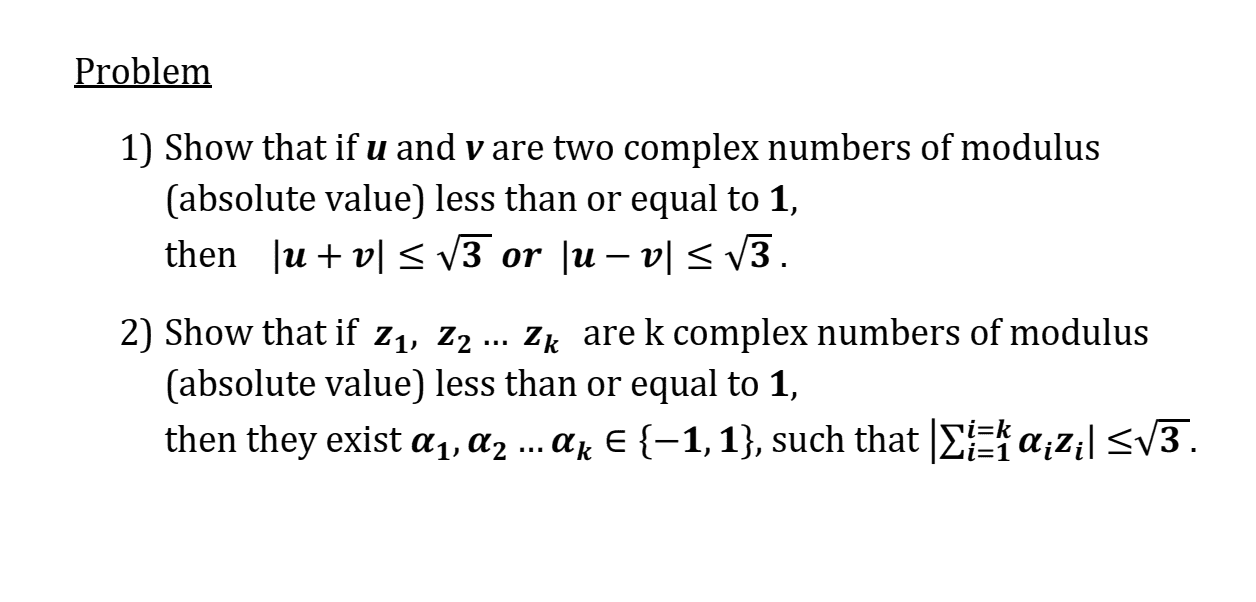# Complex numbers such that modulus (absolute value) less than or equal to 1.

• MHB
• Taleb

#### TalebWrite u= a+ bi and v= c+ di. If modulus u and v are both less than 1 the $\sqrt{a^2+ b^2}< 1$ and $\sqrt{c^2+d^2}< 1$ so $a^2+ b^2< 1$ and $c^2+ d^2< 1$.

u+ v= (a+ c)+(b+ d)i. $|u+v|= \sqrt{(a+ c)^2+ (b+ d)^2}=$$\sqrt{a^2+ 2ac+ c^2+ b^2+ 2bd+ d^2}=$$ \sqrt{(a^2+ b^2)+ (c^2+ d^2)+ (2ac+2bd)}< \sqrt{1+ 1+ 2(ac+ bd)}< \sqrt{2+ 2(ac+ bs)}$

Can you prove that $ac+ bd$ is less than 1/2?

Following up on Country Boy's calculation, notice that if $v$ is replaced by $-v$ then $b$ becomes $-b$ and $d$ becomes $-d$. Therefore $$|u+v| \leqslant \sqrt{2+2(ac+bd)}, \qquad |u-v| \leqslant \sqrt{2-2(ac+bd)}.$$ It follows that if $ac+bd>0$ then $|u-v| \leqslant\sqrt2$, and if $ac+bd<0$ then $|u+v|\leqslant\sqrt2$. That proves 1). (In fact it proves a stronger result, with $\sqrt2$ instead of $\sqrt3$.)

Problem 2) seems to be a lot harder. I found a sketch here of how to prove it by induction (again with $\sqrt2$ rather than $\sqrt3$).